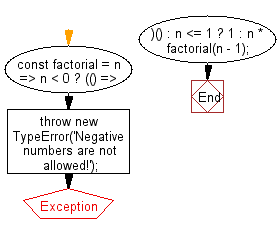# JavaScript: Calculate the factorial of a number

## JavaScript fundamental (ES6 Syntax): Exercise-238 with Solution

Write a JavaScript program to calculate the factorial of a number.

• Use recursion.
• If n is less than or equal to 1, return 1.
• Otherwise, return the product of n and the factorial of n - 1.
• Throw a TypeError if n is a negative number.

Sample Solution:

HTML Code:

``````<!DOCTYPE html>
<html>
<meta charset="utf-8">
<title>Calculate the factorial of a number</title>
<body>

</body>
</html>
```
```

JavaScript Code:

``````//#Source https://bit.ly/2neWfJ2
const factorial = n =>
n < 0
? (() => {
throw new TypeError('Negative numbers are not allowed!');
})()
: n <= 1
? 1
: n * factorial(n - 1);

console.log(factorial(1));
console.log(factorial(5));
console.log(factorial(7));
```
```

Sample Output:

```1
120
5040
```

Pictorial Presentation:Flowchart:Live Demo:

See the Pen javascript-basic-exercise-238-1 by w3resource (@w3resource) on CodePen.

Improve this sample solution and post your code through Disqus

What is the difficulty level of this exercise?

Test your Programming skills with w3resource's quiz.

﻿

## JavaScript: Tips of the Day

How to check whether a string contains a substring in JavaScript?

ECMAScript 6 introduced String.prototype.includes:

```const string = "foo";
const substring = "oo";

console.log(string.includes(substring));
```

includes doesn't have Internet Explorer support, though. In ECMAScript 5 or older environments, use String.prototype.indexOf, which returns -1 when a substring cannot be found:

```var string = "foo";
var substring = "oo";

console.log(string.indexOf(substring) !== -1);
```

Ref: https://bit.ly/3fFFgZv